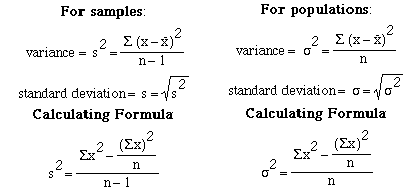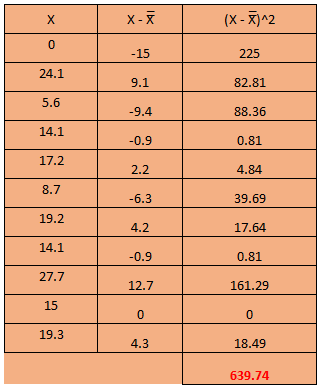### Variance and Standard Deviation

In “Range, Interquartile Range and Box Plot” section, it is explained that Range, Interquartile Range (IQR) and Box plot are very useful to measure the variability of the data.

There are two other kind of variability that a statistician use very often for their study.

1. Variance
2. Standard Deviation

#### Why variance and Standard Deviation are good measures of variability?

Because variance and standard deviation consider all the values of a variable to calculate the variability of your data.

There are two types of variance and standard deviation in terms of Sample and Population. First their formula has been given. Then, what is the difference between sample and population has been discussed below.

#### Variance:

Here is the formula for sample and population variance and standard deviation. There is slight difference observe them carefully.Where

• X is individual one value
• N is size of population
• x̄ is the mean of population

#### How to calculate variance step by step:

1. Calculate the mean x̄.
2. Subtract the mean from each observation. X- x̄
3. Square each of the resulting observations. (X- x̄) ^2
4. Add these squared results together.
5. Divide this total by the number of observations n (in case of population) to get variance S2. If you are calculating sample variance then divide by n-1.
6. Use the positive square root to get standard deviation S.Here,

N =11

N-1=10

Mean (x̄) =15

Sample variance ( s² ) = 639.74/10 = 63.97

Population ( σ² ) = 639.74/11 = 58.16

S = 8.00

σ  = 7.6

#### Intuition:

1. If variance is high, that means you have larger variability in your dataset. In the other way, we can say more values are spread out around your mean value.
2. Standard deviation represents the average distance of an observation from the mean
3. The larger the standard deviation, larger the variability of the data.

#### Standard Deviation:

The Standard Deviation is a measure of how spread out numbers are. Its symbol is σ (the greek letter sigma) for population standard deviation and S for sample standard deviation. It is the square root of the Variance.

#### Population vs. Sample Variance and Standard Deviation

The primary task of inferential statistics (or estimating or forecasting) is making an opinion about something by using only an incomplete sample of data.

In statistics, it is very important to distinguish between population and sample. A population is defined as all members (e.g. occurrences, prices, annual returns) of a specified group. Population is the whole group.

A sample is a part of a population that is used to describe the characteristics (e.g. mean or standard deviation) of the whole population. The size of a sample can be less than 1%, or 10%, or 60% of the population, but it is never the whole population. As both sample and population are not same thing therefore slight difference is there in their formula.

##### A question may raise that at the time of calculating Variance why we do square the difference?

To get rid of negatives so that negative and positive don’t cancel each other when added together.

+5 -5 = 0